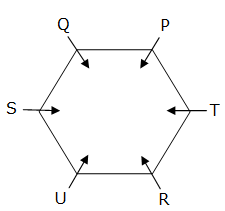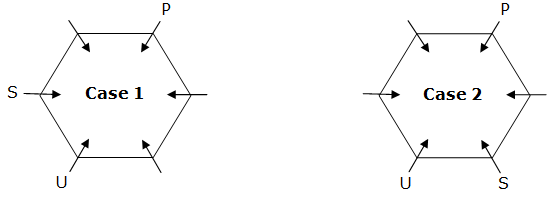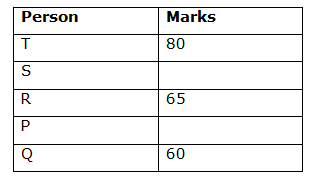# SBI Clerk Prelims Reasoning Ability Questions 2020 (Day-04)

Dear Aspirants, Our IBPS Guide team is providing new series of Reasoning Questions for SBI Clerk Prelims 2020 so the aspirants can practice it on a daily basis. These questions are framed by our skilled experts after understanding your needs thoroughly. Aspirants can practice these new series questions daily to familiarize with the exact exam pattern and make your preparation effective.

[WpProQuiz 7612]

Ensure Your Ability Before the Exam – Take SBI Clerk 2020 Prelims Free Mock Test

Seating Arrangement

Directions (1-5): Study the following information carefully and answer the questions given below.

Six persons P, Q, R, S, T, U are seated around a hexagonal table. Each person is seated at the corner of the hexagon and all of them are facing towards the centre of the table. Two persons sit between U and P either in clockwise or anti-clockwise direction. Only one person sits between P and S. Q sits second to the right of T. R is not an immediate neighbor of Q.

1) What is the position of R with respect to U?

a) Immediate left

b) Immediate right

c) Second to the right

d) Second to the left

e) None of these

2) Which of the following persons sit exactly opposite to T?

a) U

b) R

c) P

d) S

e) Q

3) How many persons are seated between P and Q, when counted from the left of P?

a) None

b) One

c) Two

d) Three

e) More than three

4) Who sits exactly between R and P, when counted from left of P?

a) Q

b) S

c) T

d) U

e) None of these

5) Who sits at the immediate right of S?

a) Q

b) U

c) P

d) T

e) R

Inequality

Directions (6-8): In each of the following questions, relationship between different elements is shown in the statements followed by two conclusions. Find the conclusion which is definitely true.

a) If only conclusion I follows.

b) If only conclusion II follows.

c) If either conclusion I or II follows.

d) If neither conclusion I nor II follows.

e) If both conclusions I and II follow.

6) Statements:

P ≤ Q < R = S ≥ T

Conclusions:

I) P ≤ T

II) T ≤ R

7) Statements:

V = E ≥ B > M < N

Conclusions:

I) B < V

II) V = B

8) Statements:

F ≥ L = U < I ≤ D

Conclusions:

I) F < I

II) D < I

Order and Ranking

Directions (9-10): Study the following information carefully and answer the questions given below.

Five students P, Q, R, S, T wrote exams and got different marks in the examination. P scored more marks than Q but less than R, who scored 65 marks. S scored less marks than only T. The one who scored the minimum marks scored 60 marks and the one who scored the maximum marks scored 80 marks.

9) Who scored the second highest marks?

a) P

b) Q

c) R

d) S

e) T

10) Who is most likely to have scored 62 marks?

a) R

b) P

c) S

d) Q

e) T

Directions (1-5):Two persons sit between U and P either in clockwise or anti-clockwise direction. Only one person sits between P and S.Q sits second to the right of T. R is not an immediate neighbor of Q.Since R is placed next to Q, Case 2 gets cancelled.

Directions (6-8) :

I) P ≤ T (P ≤ Q < R = S ≥ T) -> False

II) T ≤ R (R = S ≥ T) -> True

So, only conclusion II follows.

I) B < V (V = E ≥ B)

II) V = B (V = E ≥ B)

So, either conclusion I or II follows.

I) F < I (F ≥ L = U < I) -> False

II) D < I (I ≤ D) -> False

So, neither conclusion I nor II follows.

Directions (9-10):